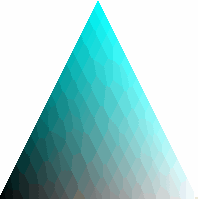Publication date: 11/10/2021

The shading model of a polygon is set using the Shade Model command.

`Shade Model (mode)`

where mode can be SMOOTH (the default) or FLAT. SMOOTH shading interpolates the colors of the primitive from one vertex to the next. FLAT mode duplicates the color of one vertex across the entire primitive.

The following script changes the color at each of a triangle’s vertices. The FILL shade model interpolates the color of the interior automatically.

`scene = Scene Box( 200, 200 ); // make a scene box...holds an OpenGL scene.`
` `
`New Window( "Shade Model", scene ); // put the scene in a window`
`scene << Clear;`
`scene << Ortho2D( -1, 1, -1, 1 );`
` `
`scene << Shade Model( SMOOTH );`
`scene << Polygon Mode( FRONT_AND_BACK, FILL );`
`scene << Begin( TRIANGLES );`
`scene << Color( 0, 0, 0 ); // black`
`scene << Vertex( -1, -1, 0 );`
`scene << Color( 0, 1, 1 ); // cyan`
`scene << Vertex( 0, 1, 0 );`
`scene << Color( 1, 1, 1 ); // white`
`scene << Vertex( 1, -1, 0 );`
`scene << End();`
` `
`scene << Update;`